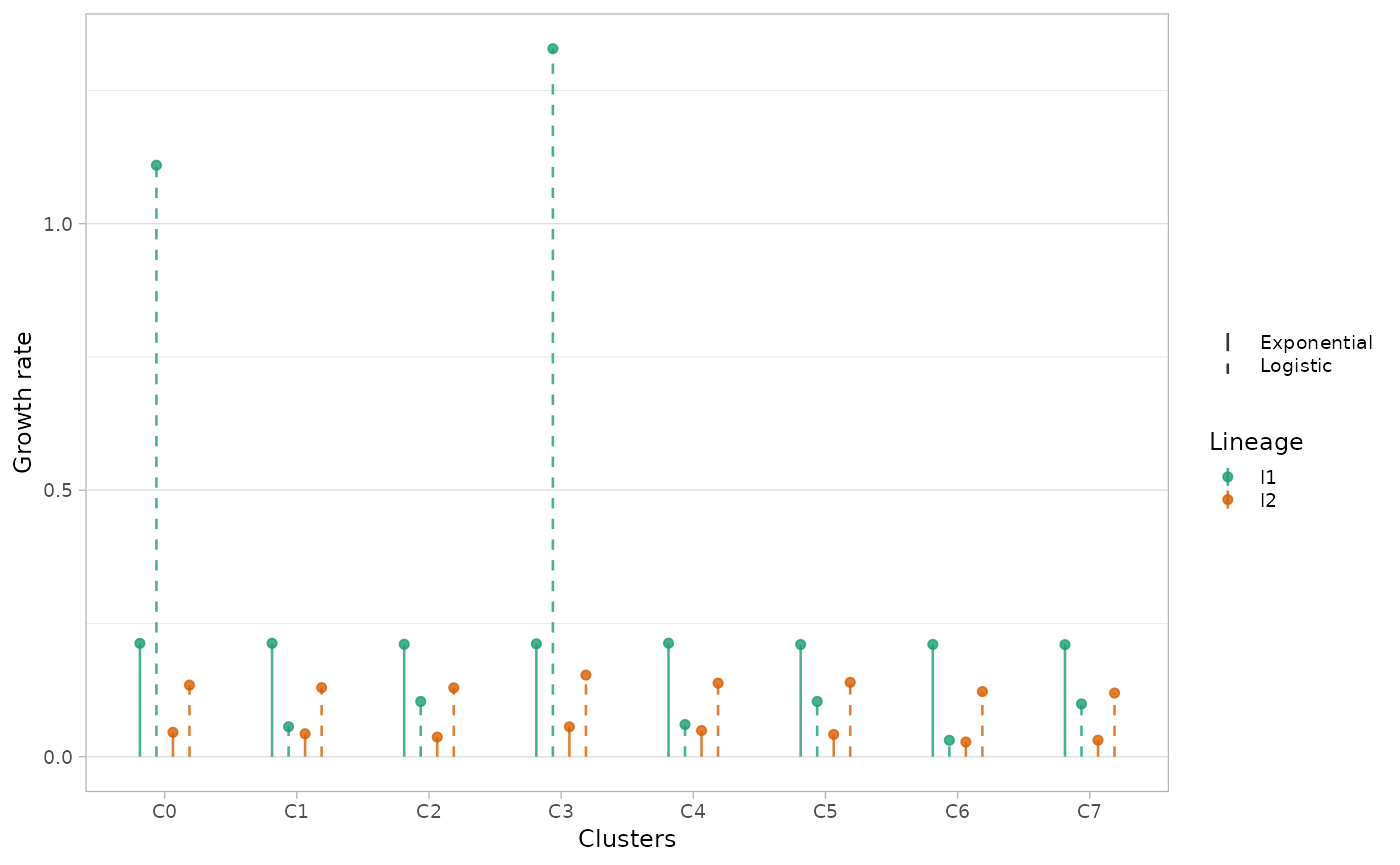library(lineaGT)
#> Warning: replacing previous import 'cli::num_ansi_colors' by
#> Warning: replacing previous import 'cli::num_ansi_colors' by
#> 'VIBER'
#> ✔ Loading VIBER, 'Variational inference for multivariate Binomial mixtures'. Support : <https://caravagn.github.io/VIBER/>
#> ✔ Loading lineaGT, 'Lineage inference from gene therapy'. Support : <https://caravagnalab.github.io/lineaGT/>
library(magrittr)
library(patchwork)
data(x.example)
x.example
#> ── [ lineaGT ]  ──── Python: /usr/share/miniconda/envs/lineagt-env/bin/python ──
#> → Lineages: l1 and l2.
#> → Timepoints: t1 and t2.
#> → Number of Insertion Sites: 66.
#>
#> ── Optimal IS model with k = 8.
#>
#>     C4 (19 ISs) : l1 [285, 209]; l2 [ 51, 492]
#>     C1 (15 ISs) : l1 [245, 177]; l2 [ 23, 289]
#>      C0 (6 ISs) : l1 [145, 240]; l2 [ 32, 373]
#>      C2 (6 ISs) : l1 [  1, 547]; l2 [  1, 388]
#>      C3 (6 ISs) : l1 [ 92, 109]; l2 [245, 751]
#>      C5 (6 ISs) : l1 [  0, 551]; l2 [  1, 828]
#>      C6 (4 ISs) : l1 [330,  16]; l2 [ 17,  38]
#>      C7 (4 ISs) : l1 [  0, 426]; l2 [  1, 198]

## Mixture weights

The mixture weights and number of ISs per cluster can be visualized with the function plot_mixture_weights() .

plot_mixture_weights(x.example)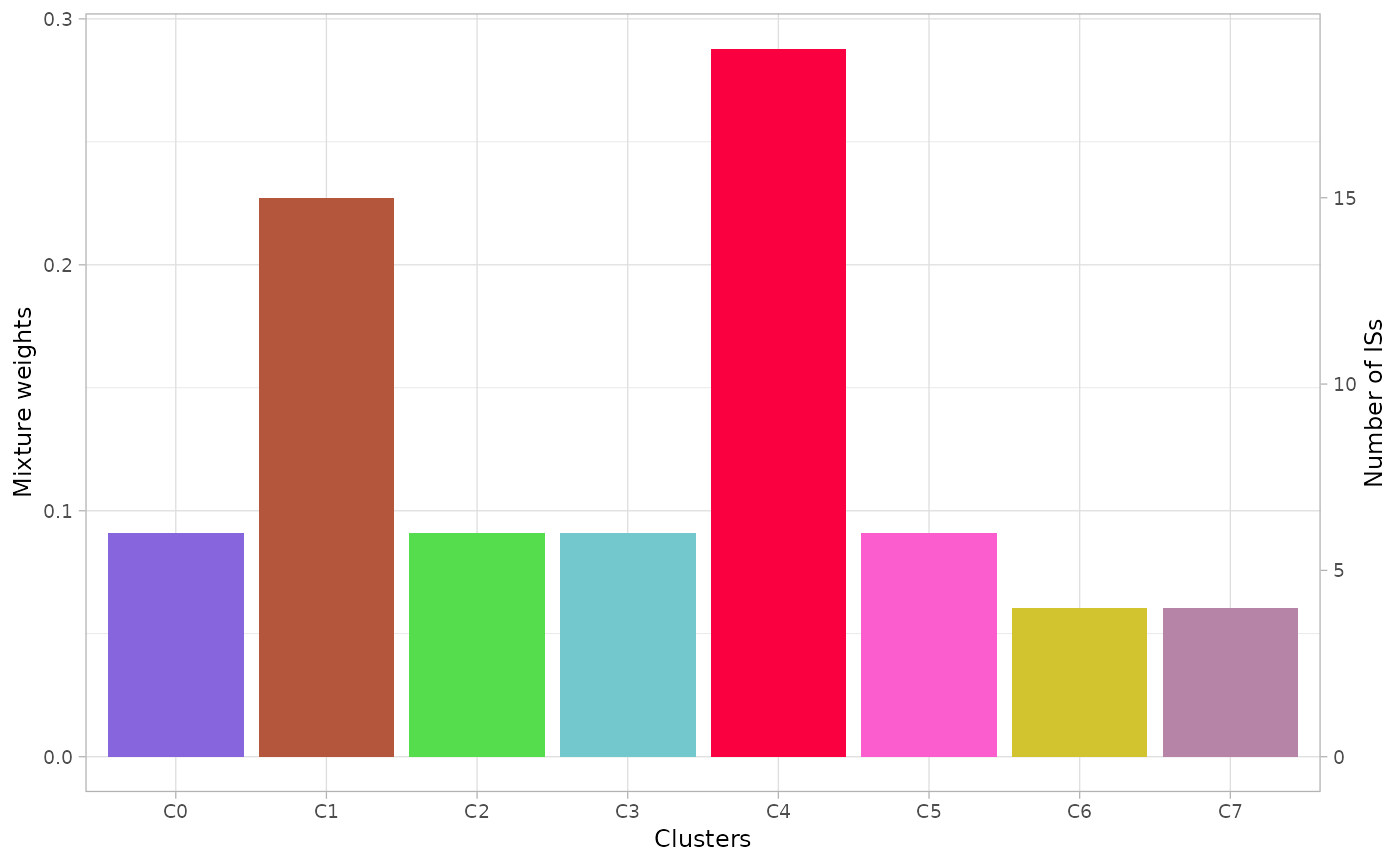## Scatterplot

The function plot_scatter_density() returns a list of 2D multivariate densities estimated by the model. The argument highlight can be used to show only a subset of clusters and the argument min_frac to show the clusters with the specified frequency in at least one dimension.

Note that the observed coverage values across lineages and over time are modeled as independent, therefore each dimension corresponds to a combination of time-point and lineage.

plots = plot_scatter_density(x.example)
plots$cov.t2.l1:cov.t1.l2 # to visualize a single plot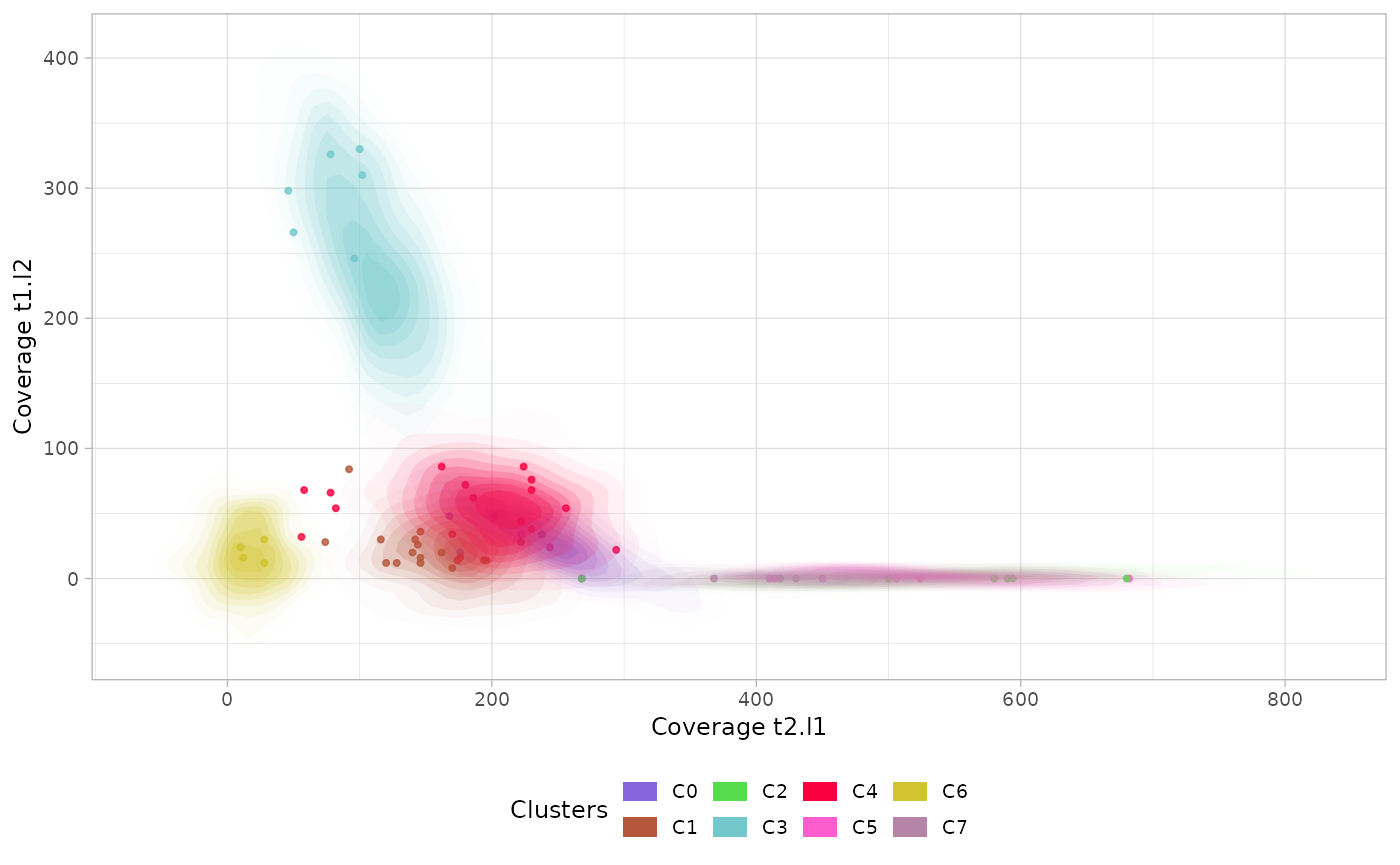## Marginal distributions The function plot_marginal() returns a plot with the marginal estimated densities for each cluster, time-point and lineage. The option single_plot returns the density of the whole mixture grouped by lineage and time-point. marginals = plot_marginal(x.example) marginals_mixture = plot_marginal(x.example, single_plot=T) patchwork::wrap_plots(marginals / marginals_mixture)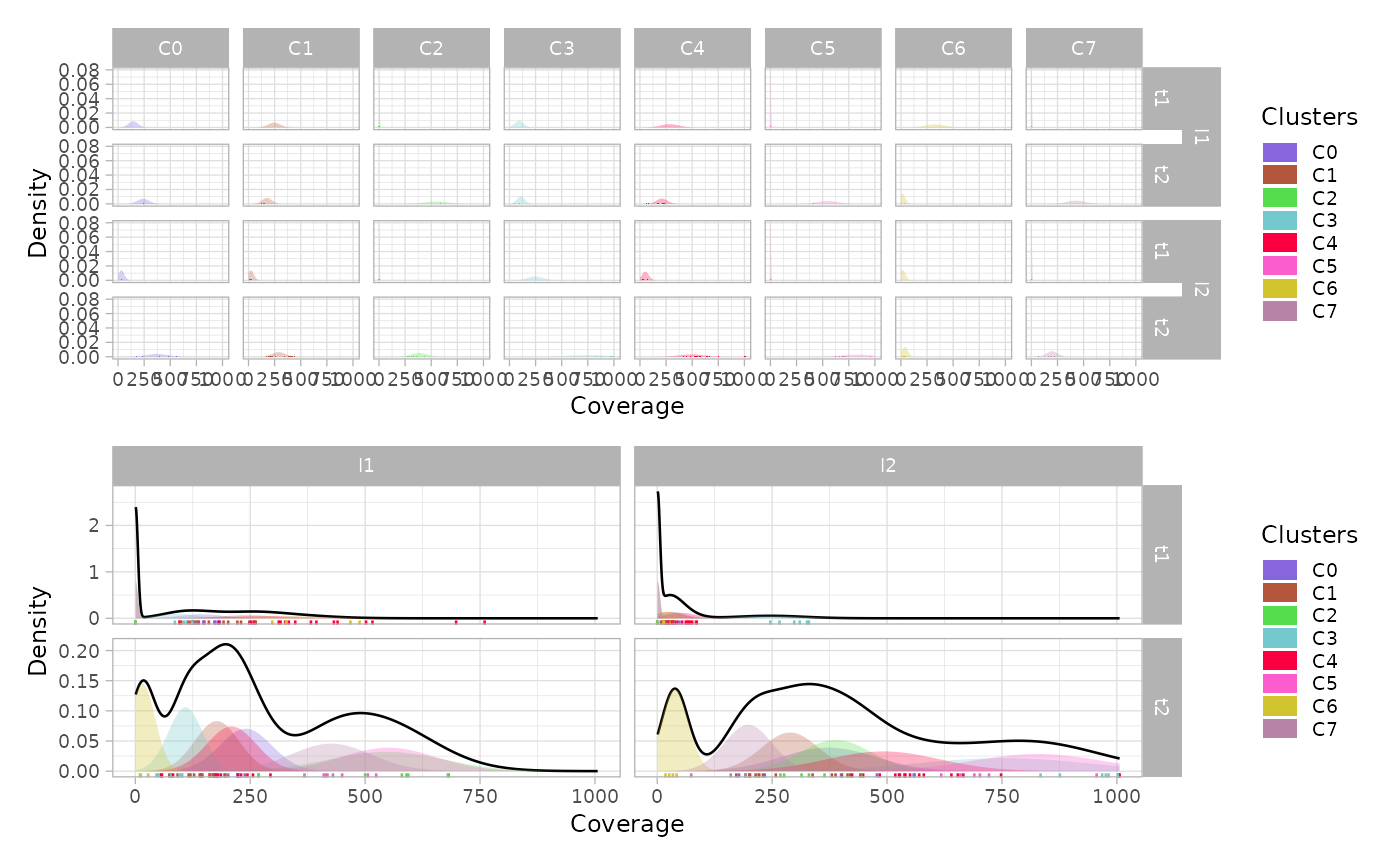## Mullerplot The function plot_mullerplot() shows the expansion of the identified populations over time. It supports the options which=c("frac","pop") corresponding to the absolule population abundance and the relative fraction, respectively. mp1 = plot_mullerplot(x.example, which="frac") mp2 = plot_mullerplot(x.example, which="pop") patchwork::wrap_plots(mp1, mp2, ncol=1)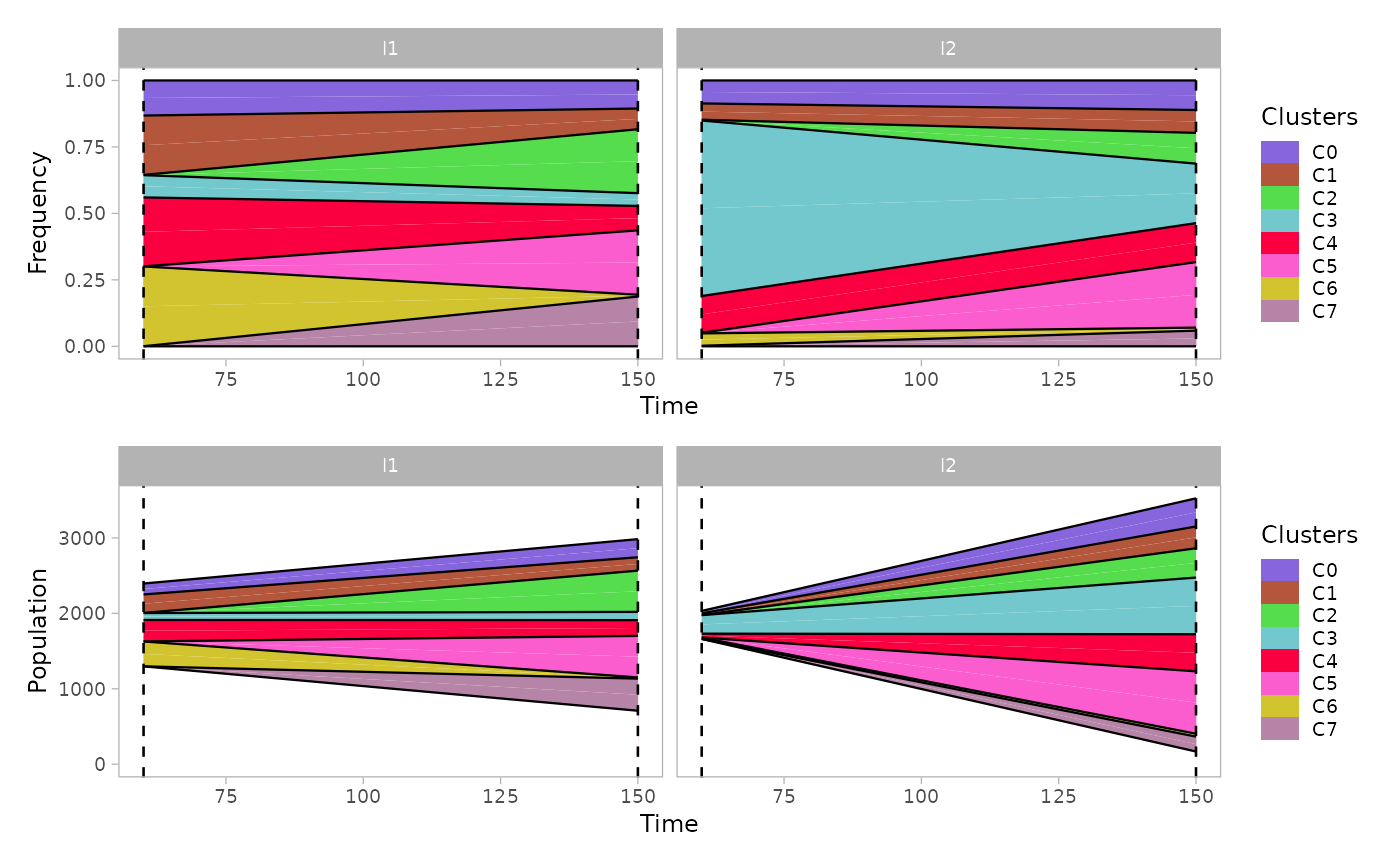If the option mutations is set to TRUE, then the subclones originated within each population will be reported as well in the mullerplot. mp1 = plot_mullerplot(x.example, which="frac", mutations=T) mp2 = plot_mullerplot(x.example, which="pop", mutations=T) patchwork::wrap_plots(mp1, mp2, ncol=1)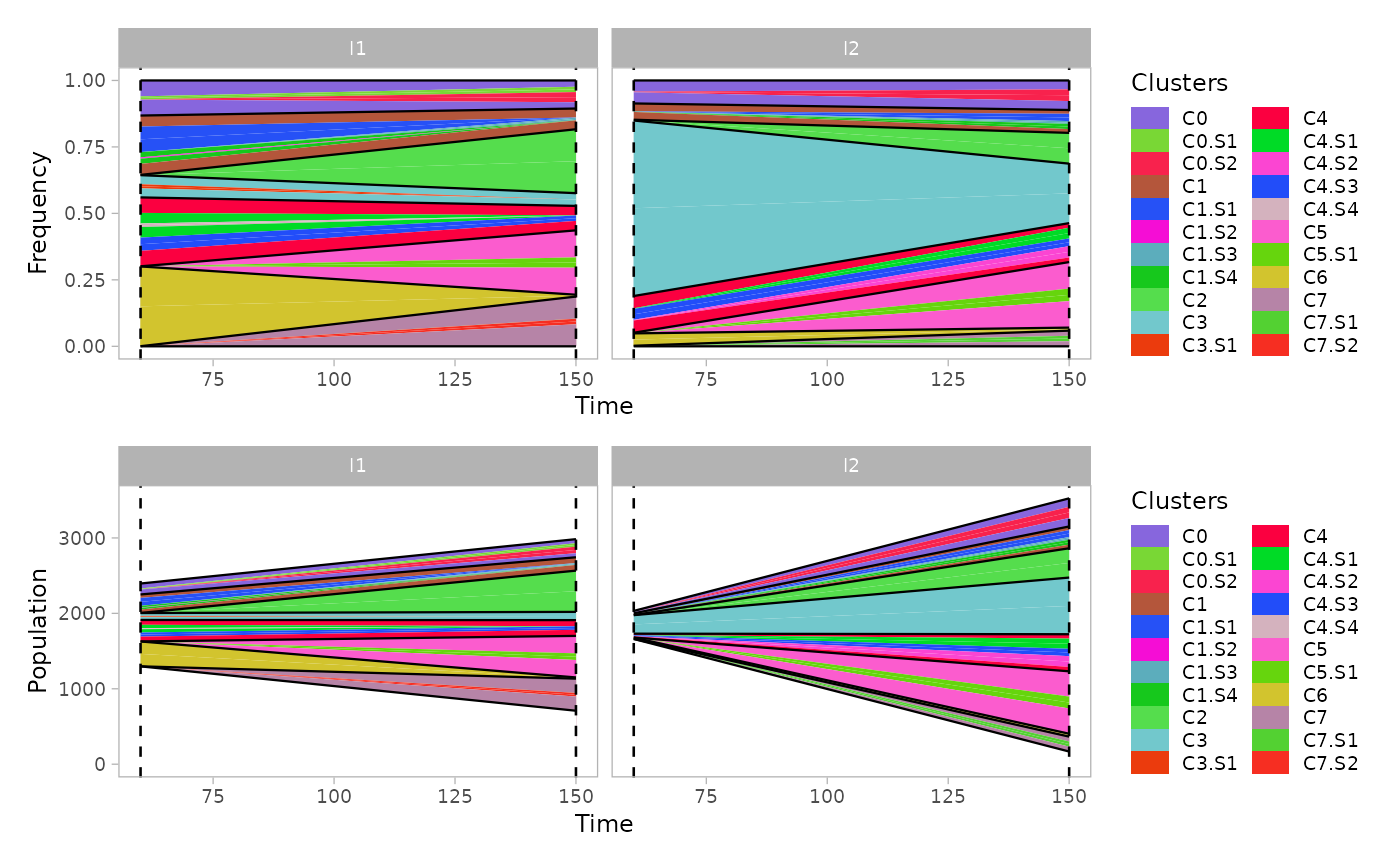The function supports also the visualization of a single clone to monitor the growth of subpopulations, through the argument single_clone. plot_mullerplot(x.example, highlight="C4", mutations=T, single_clone=T)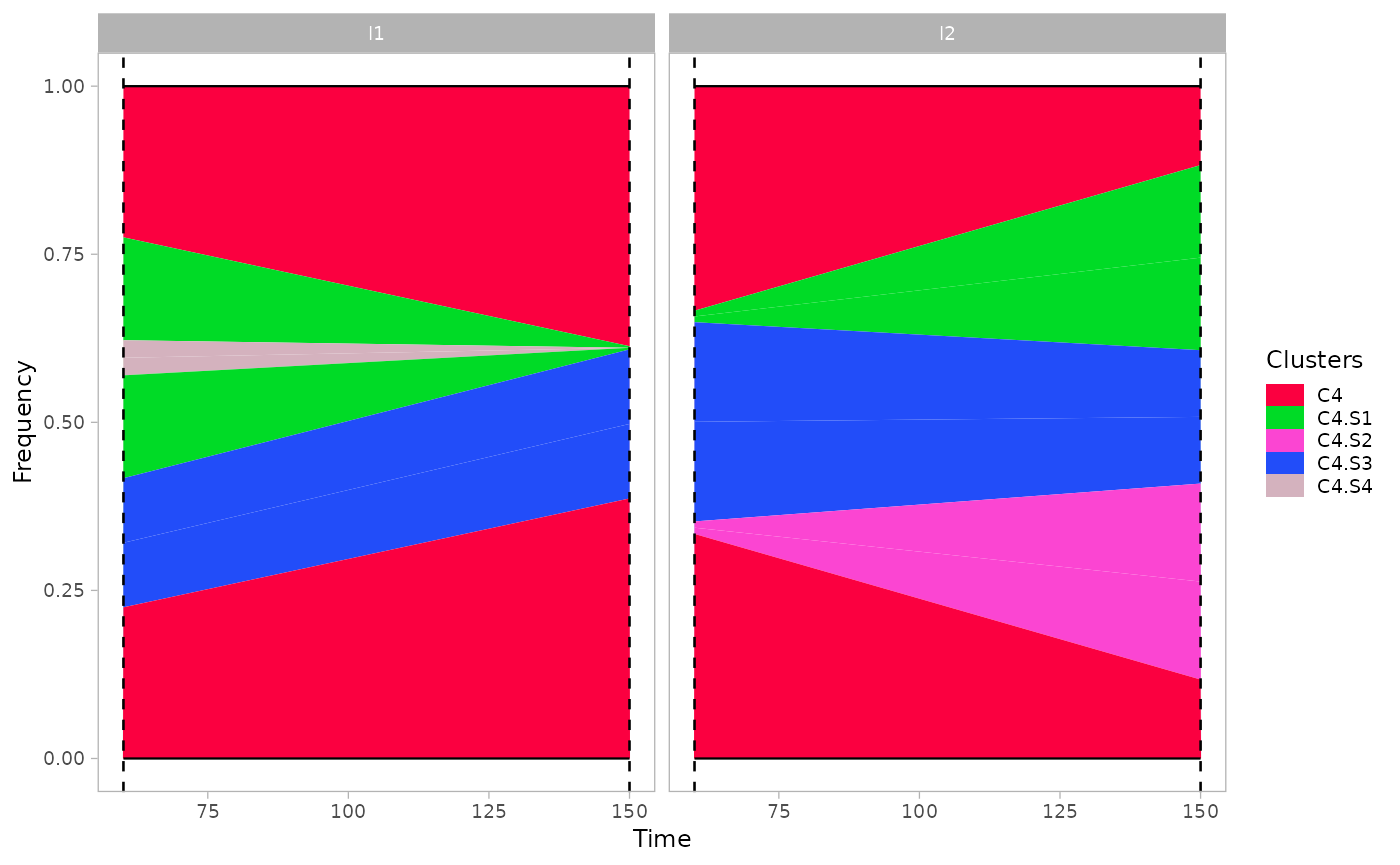Moreover, some of the identified clusters (showing low coverage in all dimensions) represents poly-clonal populations, since they cannot be uniquely identified by the mixture model. Therefore, the estimated abundance values might be readjusted according to the estimated number of populations in each clusters. estimate_n_pops(x.example) #> C0 C1 C2 C3 C4 C5 C6 C7 #> 1 2 1 1 2 1 1 1 plot_mullerplot(x.example, which="frac", mutations=T, estimate_npops=T)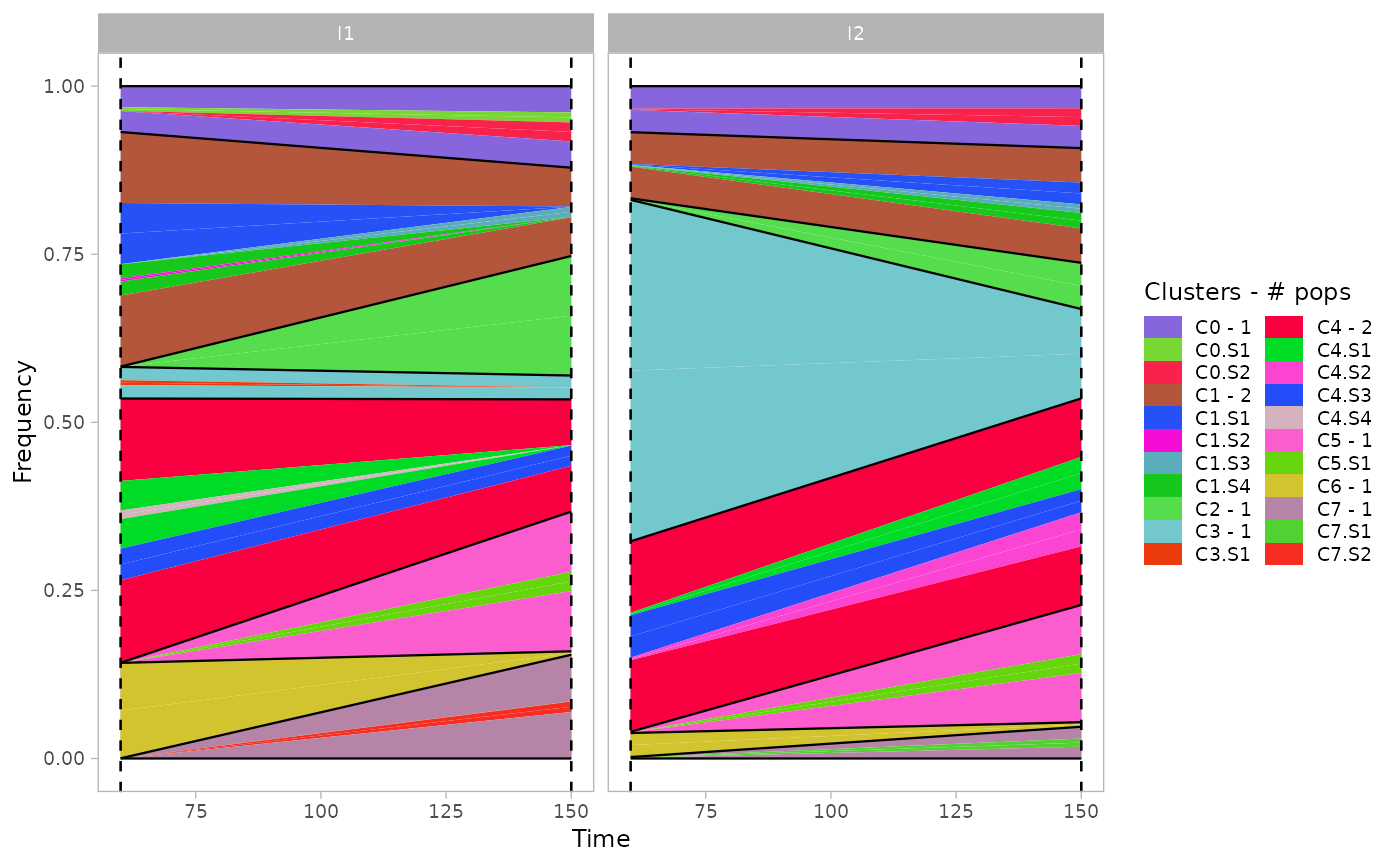## VAF The function plot_vaf_time() can be used to visualize the behaviour of mutations variant allele frequencies over time for each subclone. plot_vaf_time(x.example)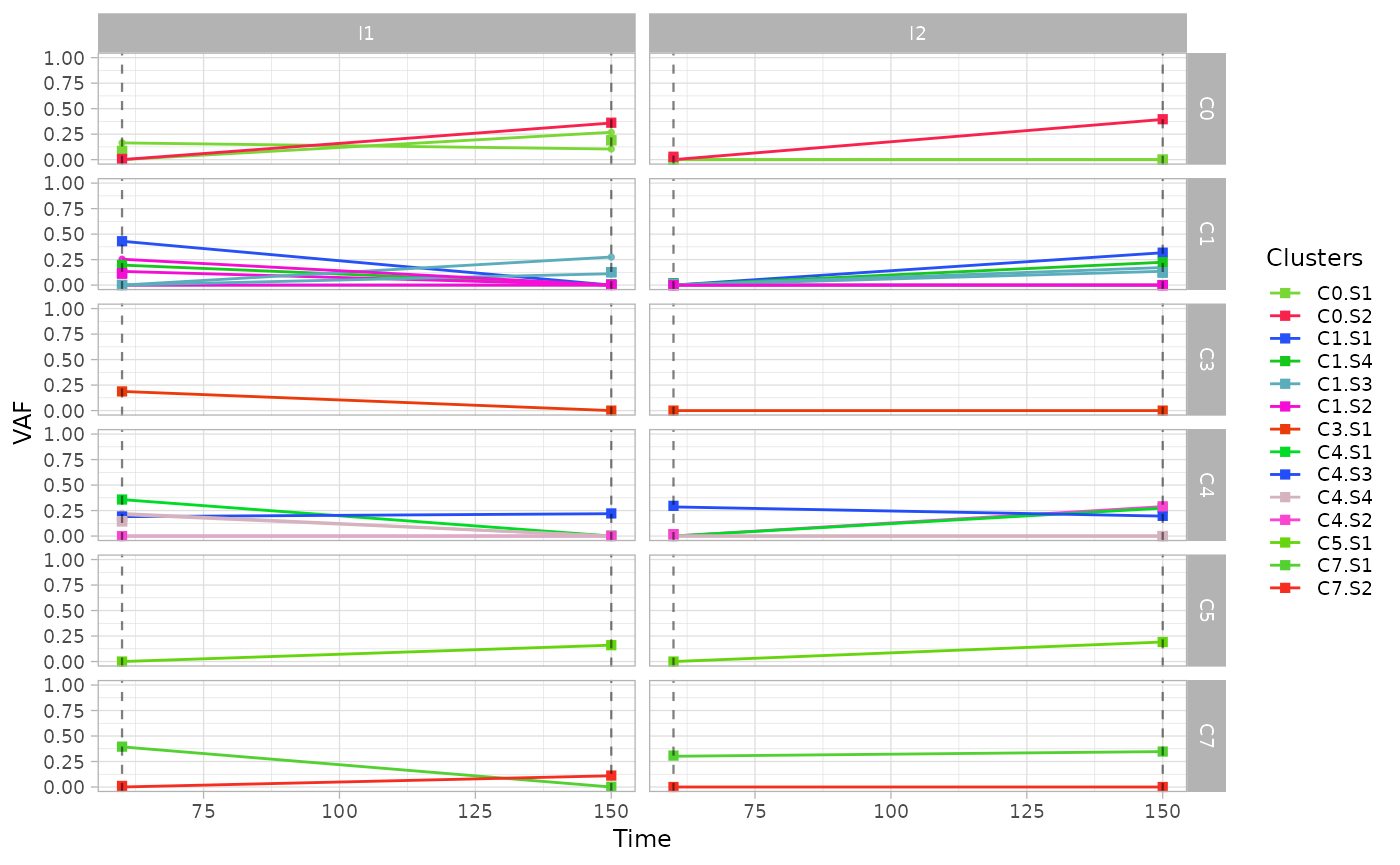## Phylogenetic evolution For each cluster of ISs, the function plot_phylogeny() reports the estimated phylogenetic tree. plot_phylogeny(x.example) #> Warning: Duplicated aesthetics after name standardisation: na.rm #> Duplicated aesthetics after name standardisation: na.rm #> Duplicated aesthetics after name standardisation: na.rm #> Duplicated aesthetics after name standardisation: na.rm #>$C0
#> Warning: Removed 1 rows containing missing values (geom_point()).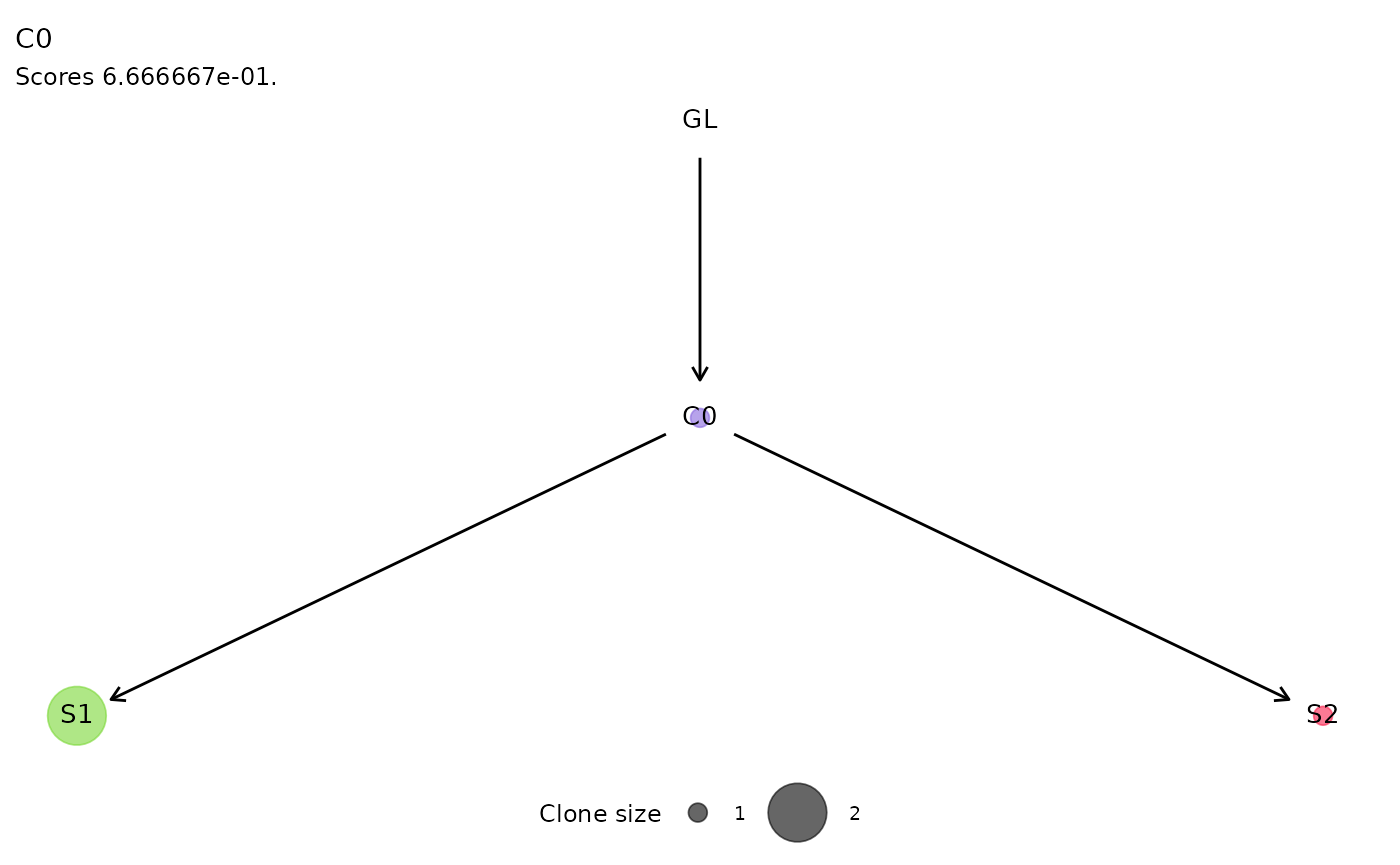#>
#> $C1 #> Warning: Removed 1 rows containing missing values (geom_point()).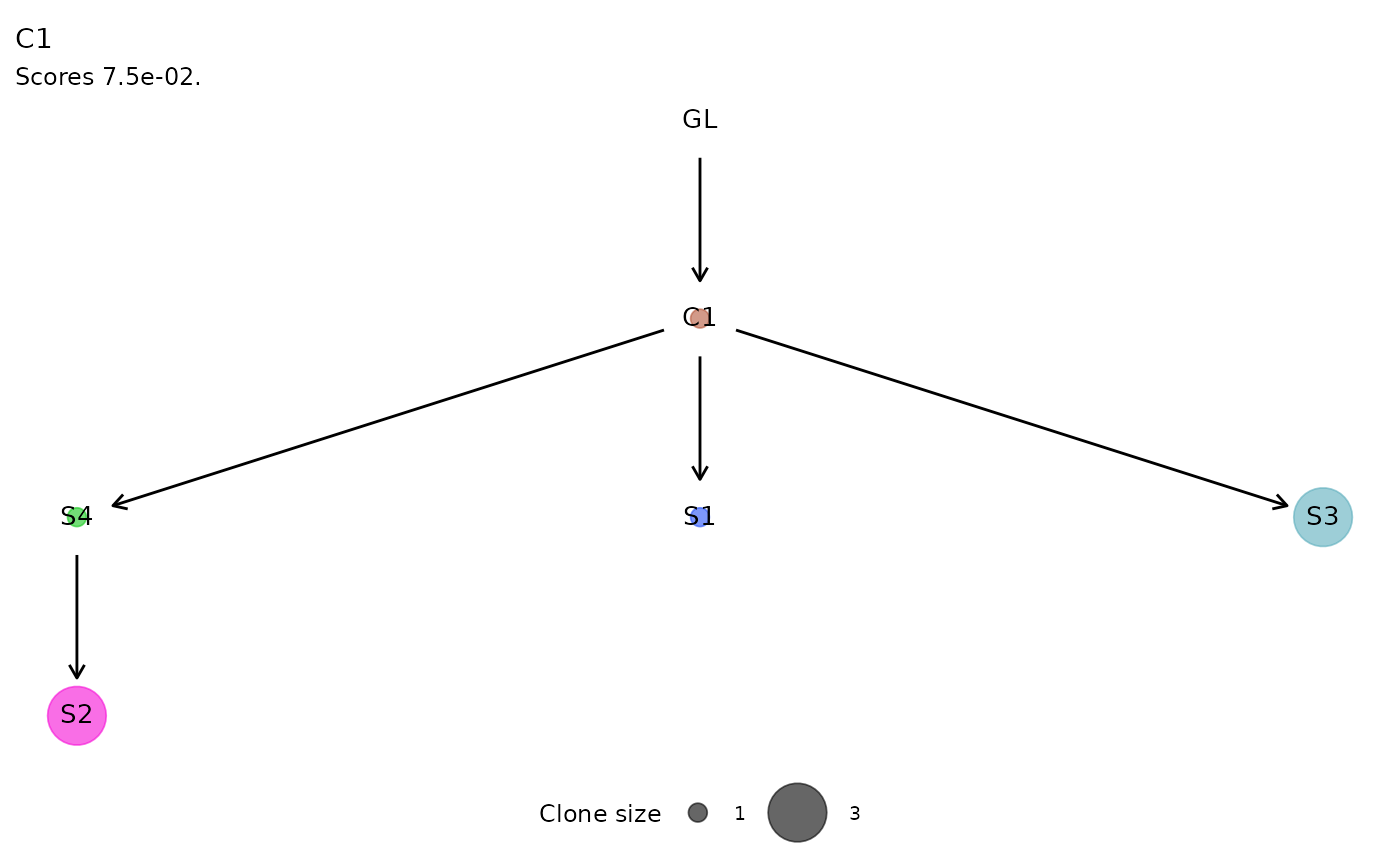#> #>$C4
#> Warning: Removed 1 rows containing missing values (geom_point()).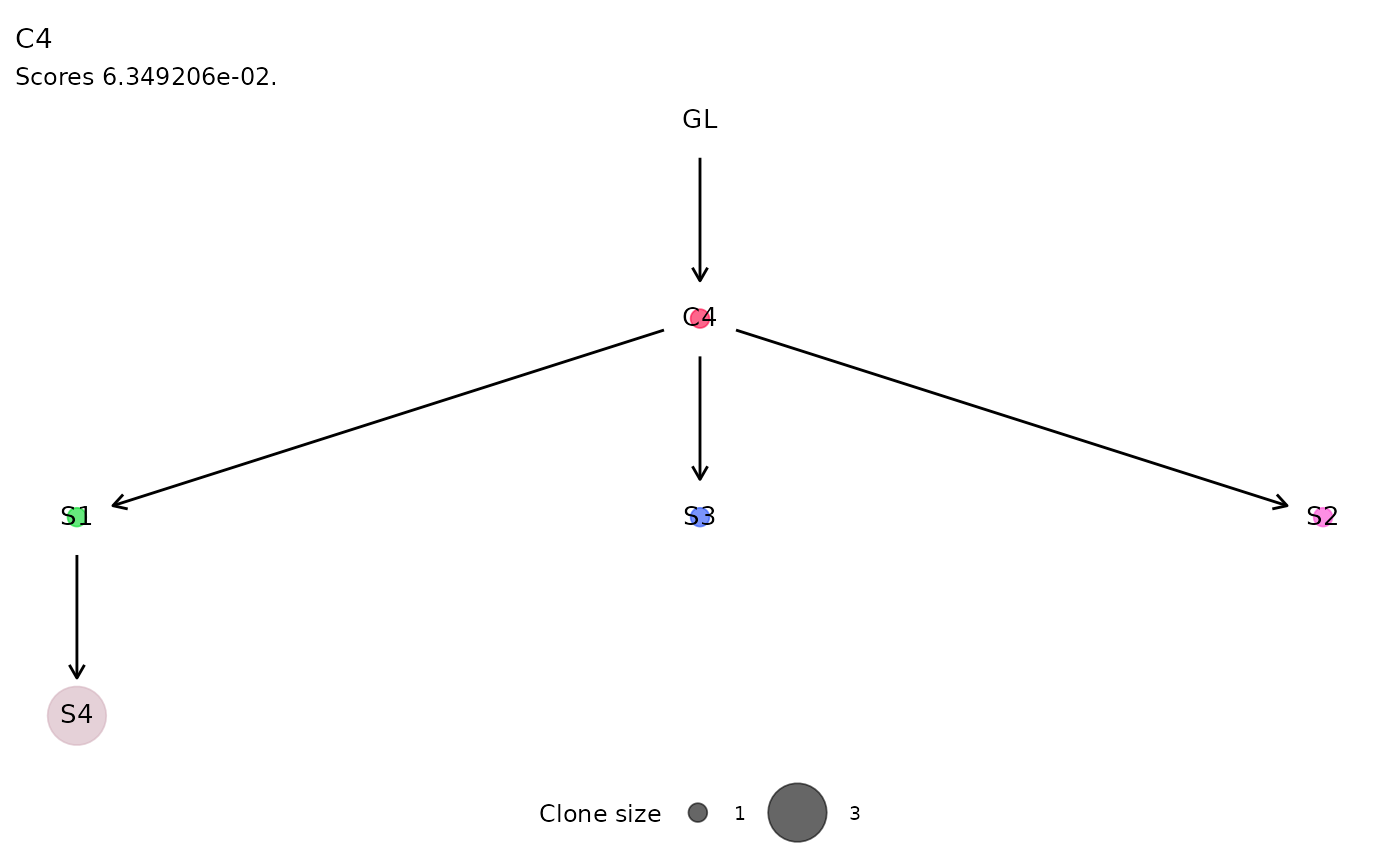#>
#> \$C7
#> Warning: Removed 1 rows containing missing values (geom_point()).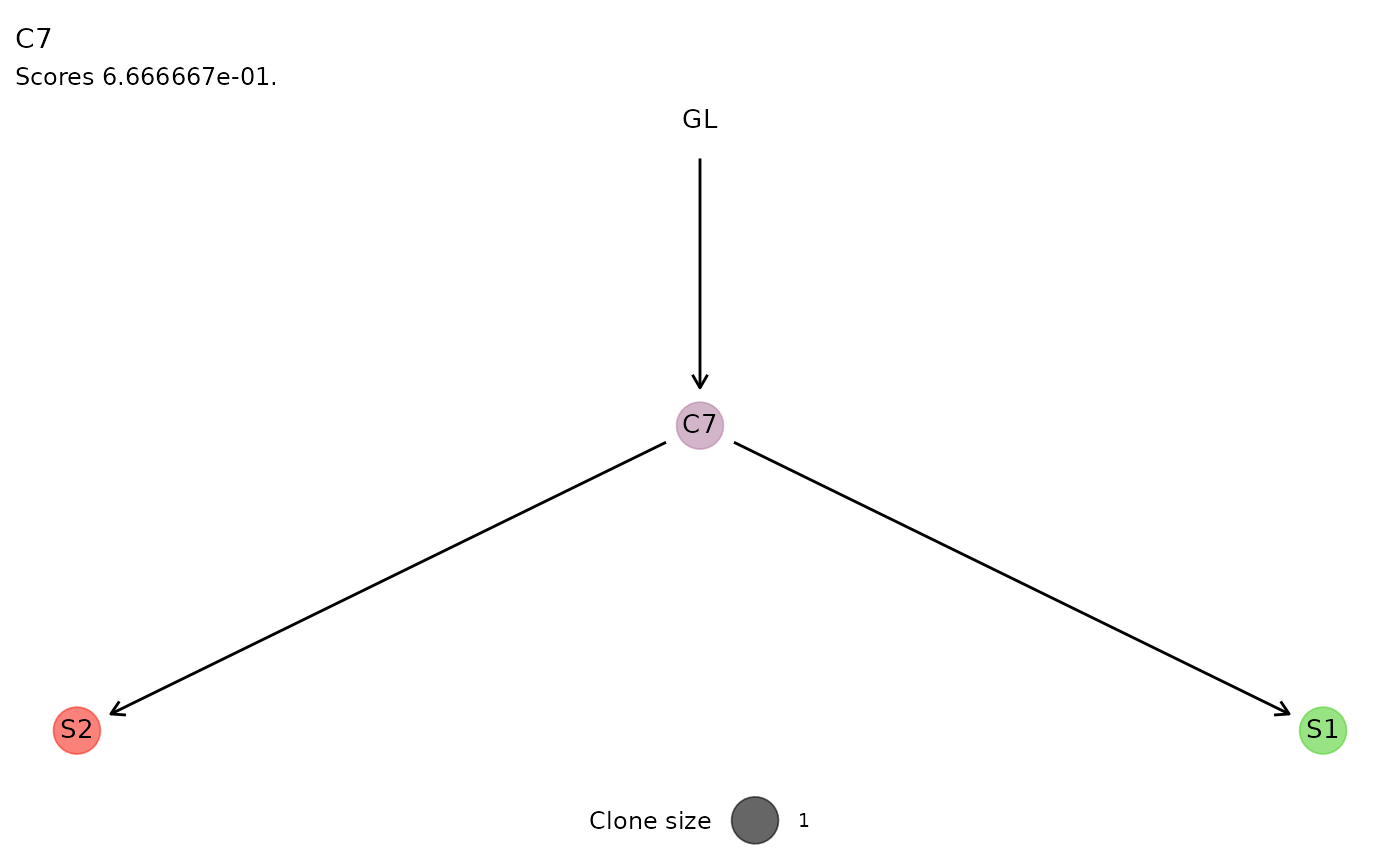## Clonal Growth

The fitted exponential and logistic growth regressions are shown with the plot_growth_regression() , reporting by default the fit of the best model, selected as the one with the highest likelihood.

Both regressions can be inspected setting show_best=F .

plot_growth_regression(x.example, show_best=F)
#> Scale for colour is already present.
#> Adding another scale for colour, which will replace the existing scale.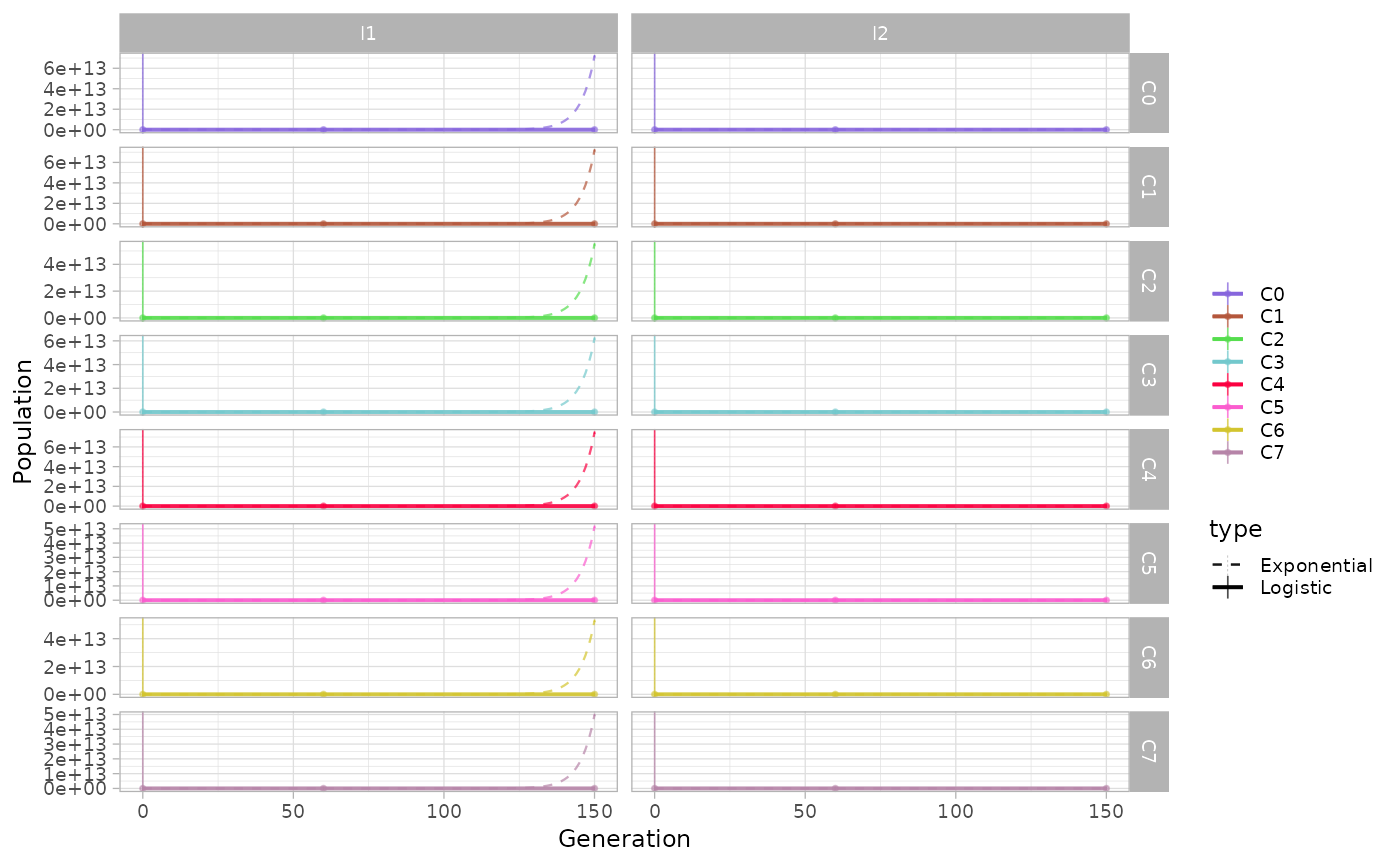The same function can be used to show the growth regressions for the subclones identified by somatic mutations.

plot_growth_regression(x.example, highlight="C4", mutations=T)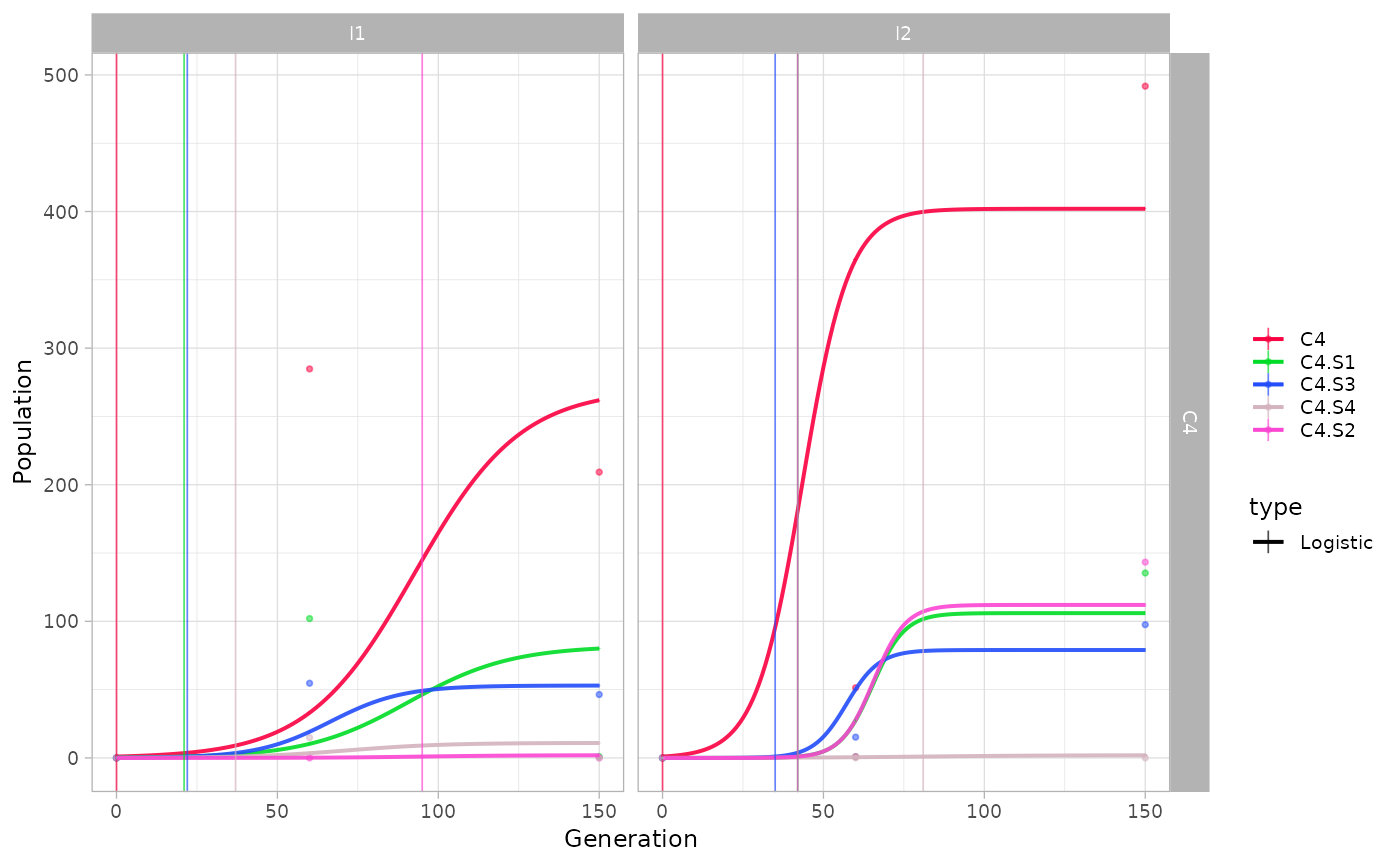An alternative way of visualising differences in growth rates is through plot_growth_rates() function, reporting the values of estimated growth rates for each (sub)population.

Disabling the show_best option, the model with lowest likelihood is shown as a dashed line.

plot_growth_rates(x.example, show_best=F)